What do you suppose happens when the vectors are in opposite directions, such as (1, 0)T and (-1, 0)T ?

The magnitude of the dot product is negative.

# Range of the Dot Product of Two Unit Vectors

Here is a sampling of bu and the dot product with au  = (1.0, 0)T for various angles.

Angle b Result Picture
000° (1.000, 0.000)T 1.000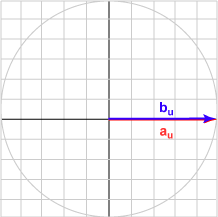015° (0.966, 0.259)T 0.966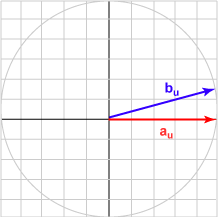030° (0.866, 0.500)T 0.866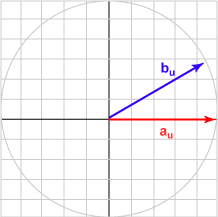045° (0.707, 0.707)T 0.707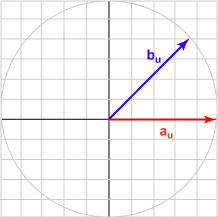060° (0.500, 0.866)T 0.500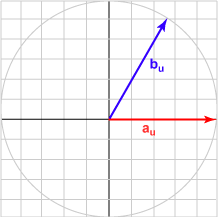075° (0.259, 0.966)T 0.259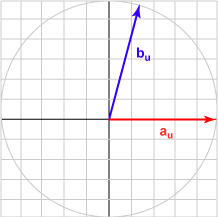090° (0.000, 1.000)T 0.000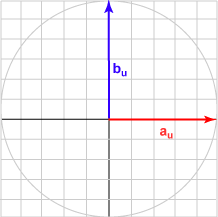105° (-0.500, 0.866)T -0.259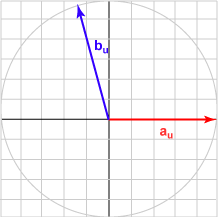120° (-0.500, 0.866)T -0.500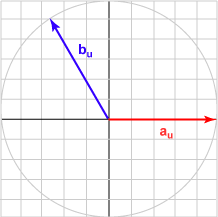135° (-0.707, 0.707)T -0.707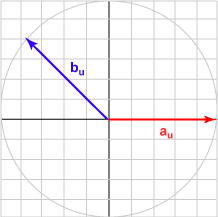150° (-0.866, 0.500)T -0.866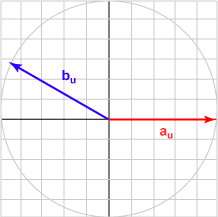165° (-0.966, 0.259)T -0.966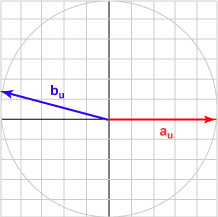180° (-1.000, 0.000)T -1.000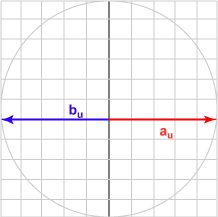The bu in each case is the unit vector represented by (cos θ, sin θ )T .

### QUESTION 6:

What do you imagine is the range of values for the dot product of the two unit vectors, au · bu  ?